Publication date: 08/13/2020

##Random Coefficient Model

The standard random coefficient model specifies a random intercept and slope for each subject. Let yij denote the measurement of the jth observation on the ith subject. Then the random coefficient model can be written as follows: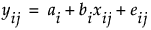where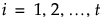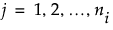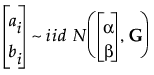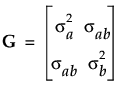and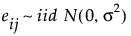You can reformulate the model to reflect the fixed and random components that are estimated by JMP as follows.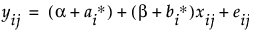where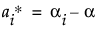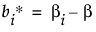and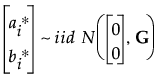with G and eij defined as above.